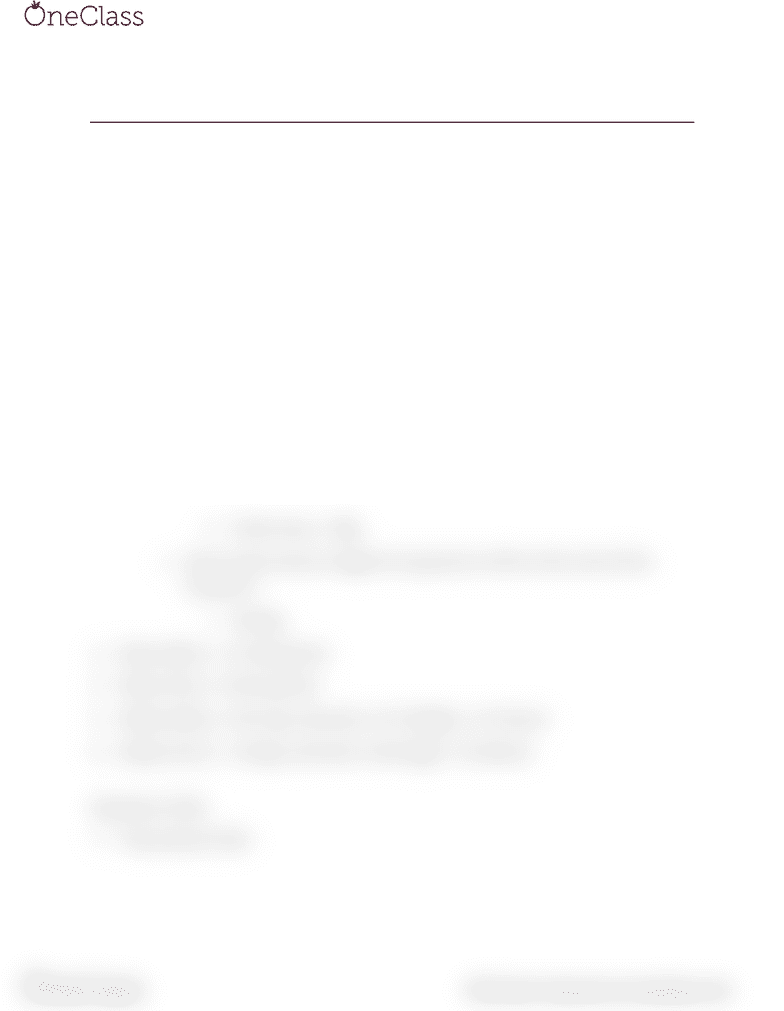Class Notes (1,100,000)
US (480,000)
TU (2,000)
CHEM (50)
Lecture 8

# CHEM 131 Lecture Notes - Lecture 8: Reagent

This preview shows half of the first page. to view the full 2 pages of the document.Chem 131 9/24
Example
1. Urea [NH2)2CO] is prepared by reacting ammonia with carbon dioxide:
a. 2•NH3(g) + CO2(g) (NH2)2 • COaq + H2O(l)
2. In one process, 637.2g of NH3 are treated with 11426 of CO2.
a. Which of the two reactants is the limiting reagent?
b. Calculate the mass of (NH2)2 CO formed.
i. N2H4•CO
1. N2 28
2. H4 4
3. C 12
4. O 16
ii. Total mass = 60g
c. How much excess reagent (in grams) is left at the end of the
reaction?
i. 318.9g
3. Mass (NH3) = 17.034 g/mol
4. Mass (CO2) = 44.01 g/mol
5. Moles (NH3) = 637.2g x [(1mol) / (17.034g)] = 37.41mol
6. Moles (CO2) = 1142g x [(1mol) / (44.01g)] = 25.95mol
Reaction Yield
7. Theoretical Yield
find more resources at oneclass.com
find more resources at oneclass.com